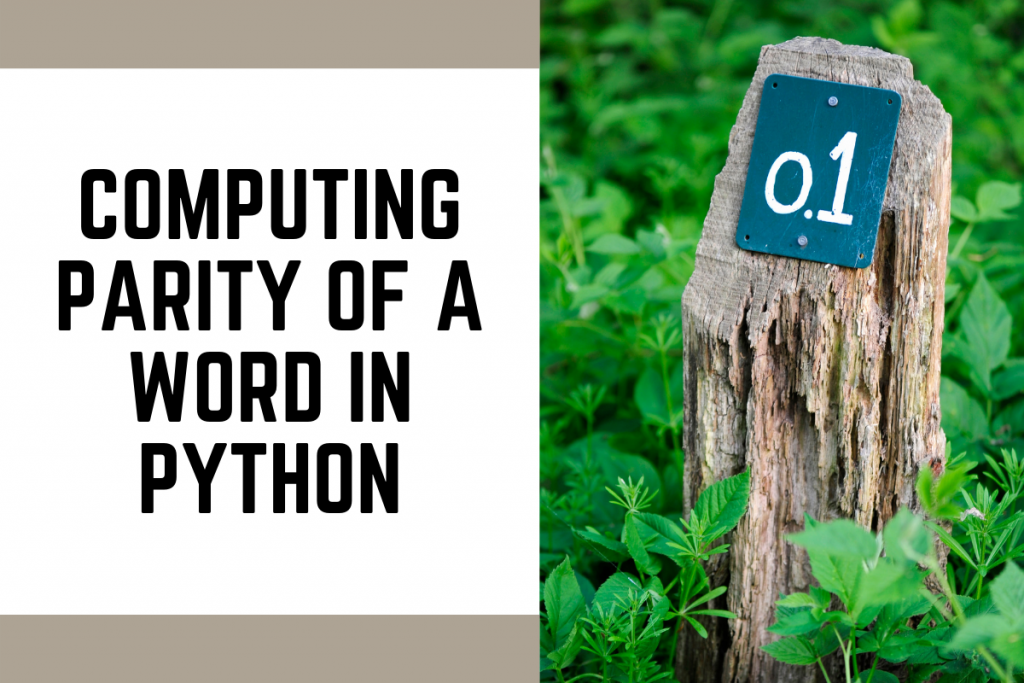# Computing Parity of a Word in PythonHello coder! So in this tutorial, we will be learning what the parity of a word is and how to calculate the parity of a word in Python programming. Let us first understand how the parity of a word is calculated.

The parity of a binary word is:

• `1` if the word contains an odd number of 1s and,
• `0` if it contains an even number of ones.

Some examples are as follows:

1. Word 1 : 1011
Number of 1s = 3
Number of 0s = 1
Parity = 1 because of the odd number of 1s.
2. Word 2 : 10001000
Number of 1s = 2
Number of 0s = 6
Parity = 0 because of the even number of 1s.

The problem statement clearly says that we need to compute the parity in a word. In simple terms, if the total number of set bits (bits with are 1) is odd, parity is `1` otherwise its `0`.

## Computing Parity of a Word in Python using the XOR operation

Method 2 will make use of the right shifting and XOR operation. The approach is implemented below and comments are added for your understanding.

```# 1. Taking Input of the word
n=int(input())
print("The word given by user is: ",n)

# parity variable initally set to 0
parity = 0

# 2.  Go through all the bits in the while loop one by one
while(n!=0):

# Check if the current LSB is 1 or 0
# if the bit is 1 then 1 and 1 => 0 otherwise 1 and 0 ==> 0

if((n&1)==1):
# XOR previous parity with 1
# It will change parity from 0 to 1 and vice versa alternately
parity^=1

# Right shift the number
n>>=1

print("Parity is: ", parity)
```

## Outputs

```The word given by user is:  1011
Parity is  1
```
```The word given by user is:  10001000
Parity is  0
```

## Conclusion

I hope you understood the problem statement and the solution very well. You can try to implement the same code on your code editors and understand more about the parity of a word.

Thank you for reading! Happy coding!